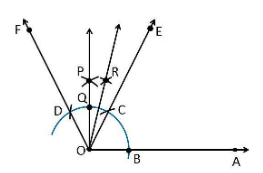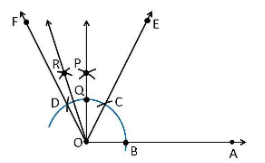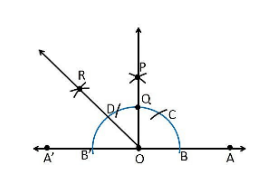Guru

# Construct the following angles and verify by measuring them by a protractor: (i) 75° (ii) 105° (iii) 135° Q.4

• 0

Yesterday i was doing the question from class 9th ncert book of math of Constructions chapter of exercise 11.1  What is the easiest way for solving it because i was not able to do this question please help me for solving this question no 4 Construct the following angles and verify by measuring them by a protractor: (i) 75° (ii) 105° (iii) 135°

Share

1. (i) 75°

Construction Procedure:

1. A ray OA is drawn.

2. With O as centre draw an arc of any radius and intersect at the point B on the ray OA.

3. With B as centre draw an arc C and C as centre draw an arc D.

4. With D and C as centre draw an arc, that intersect at the point P.

5. Join the points O and P

6. The point that arc intersect the ray OP is taken as Q.

7. With Q and C as centre draw an arc, that intersect at the point R.

8. Join the points O and R

9. Thus, ∠AOE is the required angle making 75° with OA.(ii) 105°

Construction Procedure:

1. A ray OA is drawn.

2. With O as centre draw an arc of any radius and intersect at the point B on the ray OA.

3. With B as centre draw an arc C and C as centre draw an arc D.

4. With D and C as centre draw an arc, that intersect at the point P.

5. Join the points O and P

6. The point that arc intersect the ray OP is taken as Q.

7. With Q and Q as centre draw an arc, that intersect at the point R.

8. Join the points O and R

9. Thus, ∠AOR is the required angle making 105° with OA.(iii) 135°

Construction Procedure:

1. Draw a line AOA

2. Draw an arc of any radius that cuts the line AOAat the point B and B

3. With B as centre, draw an arc of same radius at the point C.

4. With C as centre, draw an arc of same radius at the point D

5. With D and C as centre, draw an arc that intersect at the point O

6. Join OP

7. The point that arc intersect the ray OP is taken as Q and it forms an angle 90°

8. With Band Q as centre, draw an arc that intersects at the point R

9. Thus, ∠AOR is the required angle making 135° with OA.• 0